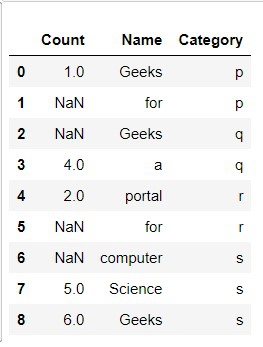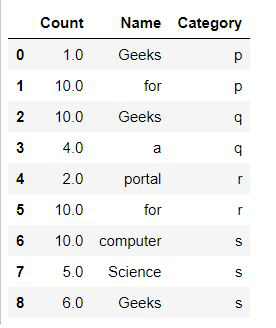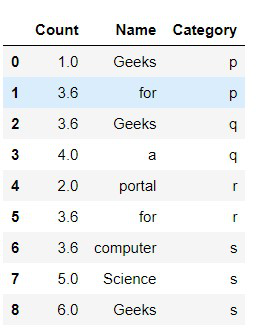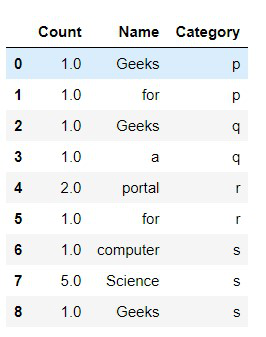# Fillna in multiple columns in place in Python Pandas

## Fillna in multiple columns in place in Python Pandas

In this article, we are going to write python script to fill multiple columns in place in Python using pandas library. A data frame is a 2D data structure that can be stored in CSV, Excel, .dB, SQL formats. We will be using Pandas Library of python to fill the missing values in Data Frame.

Let’s understand this with implementation:

First creating a Dataset with pandas

 `# Importing Required Libraries` `import` `pandas as pd` `import` `numpy as np` `# Creating a sample dataframe with NaN values` `dataframe ``=` `pd.DataFrame({``'Count'``: [``1``, np.nan,` `                                    ``np.nan, ``4``,` `                                    ``2``, np.nan,` `                                    ``np.nan, ``5``, ``6``],` `                          ` `    ``'Name'``: [``'Geeks'``,``'for'``, ``'Geeks'``,``'a'``,``'portal'``,``'for'``,` `             ``'computer'``, ``'Science'``,``'Geeks'``],` `                          ``'Category'``:``list``(``'ppqqrrsss'``)})` `# Printing The dataframe` `display(dataframe)`

Output:Example 1: Filling missing columns values with fixed values:

We can use fillna() function to impute the missing values of a data frame to every column defined by a dictionary of values.The limitation of this method is that we can only use constant values to be filled.

 `# Importing Required Libraries` `import` `pandas as pd` `import` `numpy as np` `# Creating a sample dataframe with NaN values` `dataframe ``=` `pd.DataFrame({``'Count'``: [``1``, np.nan, np.nan, ``4``, ``2``,` `                                    ``np.nan,np.nan, ``5``, ``6``],` `                          ` `    ``'Name'``: [``'Geeks'``,``'for'``, ``'Geeks'``,``'a'``,``'portal'``,``'for'``,` `             ``'computer'``, ``'Science'``,``'Geeks'``],` `                          ``'Category'``:``list``(``'ppqqrrsss'``)})` `# Creating a constant value for column Count` `constant_values ``=` `{``'Count'``: ``10``}` `dataframe ``=` `dataframe.fillna(value ``=` `constant_values)` `# Printing the dataframe` `display(dataframe)`

Output:Example 2: Filling missing columns values with mean():

In this method, the values are defined by a method called mean() which finds out the mean of existing values of the given column and then imputes the mean values in each of the missing (NaN) values.

 `# Importing Required Libraries` `import` `pandas as pd` `import` `numpy as np` `# Creating a sample dataframe with NaN values` `dataframe ``=` `pd.DataFrame({``'Count'``: [``1``, np.nan, np.nan, ``4``, ``2``,` `                                    ``np.nan,np.nan, ``5``, ``6``],` `                          ` `    ``'Name'``: [``'Geeks'``,``'for'``, ``'Geeks'``,``'a'``,``'portal'``,``'for'``,` `             ``'computer'``, ``'Science'``,``'Geeks'``],` `                          ``'Category'``:``list``(``'ppqqrrsss'``)})` `# Filling Count column with mean of Count column` `dataframe.fillna(dataframe[``'Count'``].mean(), inplace ``=` `True``)` `# Printing the Dataframe` `display(dataframe)`

Output:Example 3: Filling missing column values with mode().

The mode is the value that appears most often in a set of data values. If X is a discrete random variable, the mode is the value x at which the probability mass function takes its maximum value. In other words, it is the value that is most likely to be sampled.

 `# Importing Required Libraries` `import` `pandas as pd` `import` `numpy as np` `# Creating a sample dataframe with NaN values` `dataframe ``=` `pd.DataFrame({``'Count'``: [``1``, np.nan, np.nan,` `                                    ``1``, ``2``, np.nan,np.nan,` `                                    ``5``, ``1``],` `                          ` `    ``'Name'``: [``'Geeks'``,``'for'``, ``'Geeks'``,``'a'``,``'portal'``,``'for'``,` `             ``'computer'``, ``'Science'``,``'Geeks'``],` `                          ``'Category'``:``list``(``'ppqqrrsss'``)})` `# Using Mode() function to impute the values using fillna` `dataframe.fillna(dataframe[``'Count'``].mode()[``0``], inplace ``=` `True``)` `# Printing the Dataframe` `display(dataframe)`

Output:.

Last Updated on October 23, 2021 by admin

## Create a Pandas DataFrame from List of DictsCreate a Pandas DataFrame from List of Dicts

Pandas DataFrame is a 2-dimensional labeled data structure with columns of potentially different types. It

## Check if a column starts with given string in Pandas DataFrame?Check if a column starts with given string in Pandas DataFrame?

Check if a column starts with given string in Pandas DataFrame? In this program, we

## Pandas Series.mean()Pandas Series.mean()

Python Pandas Series.mean() Pandas series is a One-dimensional ndarray with axis labels. The labels need

## Merge two Pandas DataFrames with complex conditionsMerge two Pandas DataFrames with complex conditions

Merge two Pandas DataFrames with complex conditions In this article, we let’s discuss how to

## Add multiple columns to dataframe in PandasAdd multiple columns to dataframe in Pandas

Add multiple columns to dataframe in Pandas In Pandas, we have the freedom to add

## Reset Index in Pandas DataframeReset Index in Pandas Dataframe

Reset Index in Pandas Dataframe   Let’s discuss how to reset index in Pandas DataFrame.

## Get the substring of the column in Pandas-PythonGet the substring of the column in Pandas-Python

Get the substring of the column in Pandas-Python Now, we’ll see how we can get